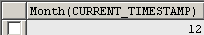Tutorials

# Mysql Date Get Month

The tutorial explains you the way to retrieve the current month.

The tutorial explains you the way to retrieve the current month.

# Mysql Date Get Month

The tutorial explains you the way to retrieve the current month.

Understand with Example

The Tutorial illustrate an example from 'Mysql Date Get Month'. To understand this example, we use Month(Current_timestamp) that return the value of the current month.

Month(Current_TimeStamp) : The Month(Current_Timestamp) returns you the current month value. This is also considered as synonyms for now( ).

Query:

The Query below return you the  current value for the month.

 Select Month(CURRENT_TIMESTAMP);

Output:-